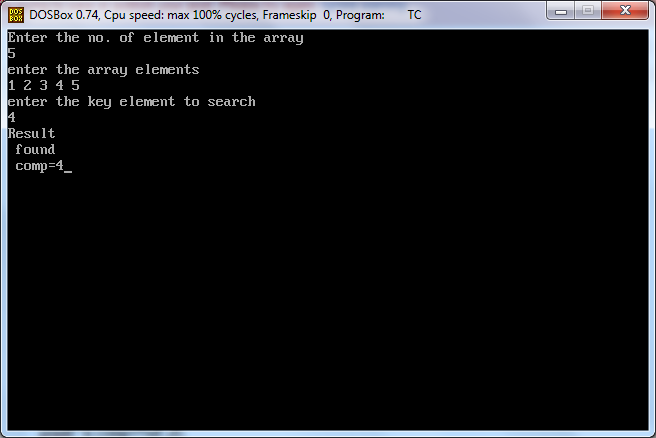## Friday, 6 September 2013

#include<stdio.h>
#include<conio.h>
void main()
{
int f=0,a,d=0,c,v=0,i,key,n;
clrscr();
printf("Enter the no. of element in the array \n");
scanf("%d",&n);
printf("enter the array elements \n");
for(i=0;i<n;i++)
scanf("%d",&a[i]);
printf("enter the key element to search");
scanf("%d",&key);
for(i=0;i<n;i++)
{d++;
if(a[i]==key)
{c[v]=a[i];
v++;
f++;
break;
}
}
if(f==0)
}
else
{printf("\n found");
}
printf("\n comp=%d",d);
getch();
}intf("Result");
if(f==0)
}
else
{printf("\n found");
}
printf("\n comp=%d",d);
getch();
}

OUTPUT:-#### 1 comment

1.Ahaa, it’s nice conversation on the subject of this post at this place, I actually have read all that, therefore at this time me additionally commenting at this place.
learn c++